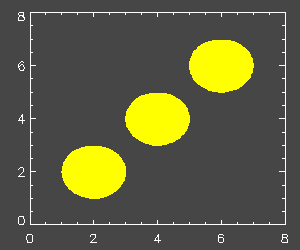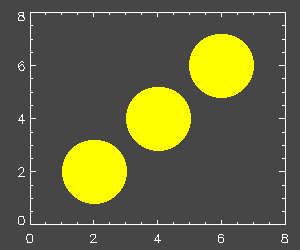# Creating a Circle in IDL

QUESTION: How can I create a circle in IDL?ANSWER: Kevin Ivory from the Max Plank Institute offers a very simple algorithm for calculating a circle in IDL:

```   FUNCTION CIRCLE, xcenter, ycenter, radius
points = (2 * !PI / 99.0) * FINDGEN(100)
x = xcenter + radius * COS(points )
y = ycenter + radius * SIN(points )
RETURN, TRANSPOSE([[x],[y]])
END
```

The Circle function returns a 2 by 100 array that can be sent to the cgPlotS command to draw open circles or to the cgColorFill command to draw closed or filled circles. The circles created with cgColorFill can be filled with different colors.

For example, type these commands:

```   Window
TVLCT, [255,0], [255,255], [0,0], 1
cgColorFill, CIRCLE(65, 65, 20), Color=1, /Device
cgPlotS, CIRCLE(130, 130, 30), /Device
cgPlotS, CIRCLE(195, 195, 20), /Device, Color=2
cgPlotS, CIRCLE(195, 195, 10), /Device, Color=1
```

You see the result in the picture below.

Notice that DEVICE coordinates were used in the code above. The Circle function can also be used with DATA coordinates, but one of the weakness of this simple algorithm is that you are not guaranteed circles when using data coordinates. In fact, if you are not careful about the aspect ratio of your plots, your circles will end up ellipses. Consider these commands:

```   Window, XSize=300, YSize=250
cgPlot, Findgen(8), /NoData, Background='charcoal', AxisColor='white'
FOR j=1,3 DO cgColorFill, CIRCLE(2*j, 2*j, 1), Color=1
```

Here is an illustration of what these commands produce.For this reason, I prefer to use the NASA Goddard Astonomy Library routine TVCIRCLE. With this routine you are guaranteed that you will see a circle on the display whether you specify the circle coordinates in data or device coordinates. You might be interested in the companion routine TVELLIPSE , too.

For example:

```   cgPlot, Findgen(8), /NoData, Background='charcoal', AxisColor='white'
FOR j=1,3 DO TVCIRCLE, 1, 2*j, 2*j, Color=1, /Data, /Fill
```

The code above with the call to TVCIRCLE produces this result:[Return to IDL Programming Tips]